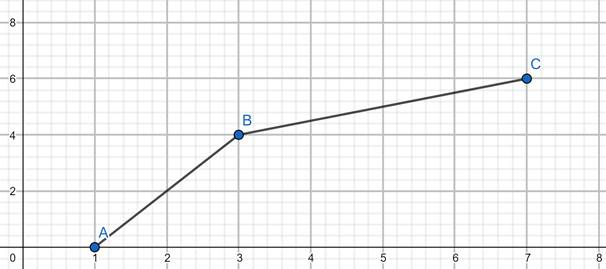# The domain and the range of the function f.### Precalculus: Mathematics for Calcu...

6th Edition
Stewart + 5 others
Publisher: Cengage Learning
ISBN: 9780840068071### Precalculus: Mathematics for Calcu...

6th Edition
Stewart + 5 others
Publisher: Cengage Learning
ISBN: 9780840068071

#### Solutions

Chapter 2, Problem 11T

a.

To determine

## The domain and the range of the function f.

Expert Solution

The domain of the function f = [0, 6]. The range of the function f = [1, 7]

### Explanation of Solution

Given:

The graph of f is given.

Concept Used:

The concept of Domain and Range is used.

Calculation:

To Calculate Domain, look at the values that x can accept.

In the graph, x lies between 0 and 6, both included.

So, the domain of graph f is [0, 6].

To Calculate Range, look at the values of y.

In the graph, ylies between 1 and 7, both included.

So, the range of graph f is [1, 7].

Conclusion:

The domain of the function f = [0, 6]. The range of the function f = [1, 7].

b.

To determine

Expert Solution

### Explanation of Solution

Given information : The graph of f is given.

Graph : The graph is plotted below.Interpretation : The graph of f1 is plotted.

c.

To determine

### The average rate of change of fbetween x = 2 and x = 6.

Expert Solution

The average rate of change of f between x = 2 and x = 6 is 54 .

### Explanation of Solution

Given:

The graph of f is given.

Concept Used:

The concept of Rate of change of graph is used.

Calculation:

To Calculate the average rate of change of f between x = 2 and x = 6.

Average Rate of Change of f = value of y at x=6 - value of y at x=262

7264=54

Conclusion:

The average rate of change of fbetween x = 2 and x = 6 is 54 .

### Have a homework question?

Subscribe to bartleby learn! Ask subject matter experts 30 homework questions each month. Plus, you’ll have access to millions of step-by-step textbook answers!